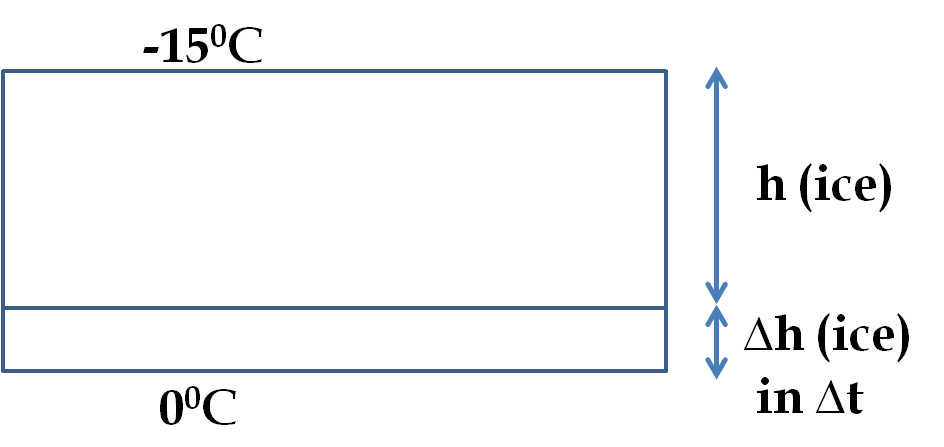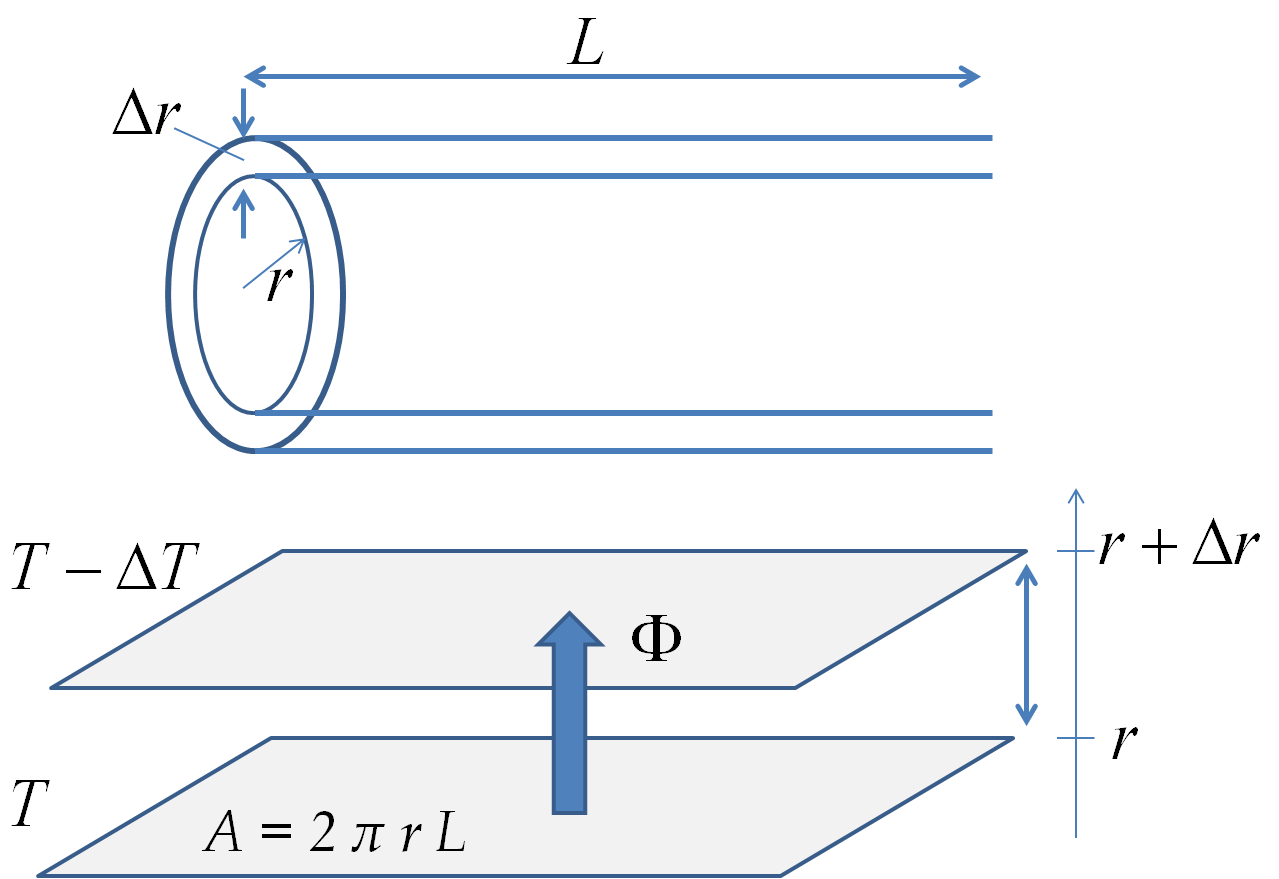## Section23.6Heat Transfer Bootcamp

### Subsection23.6.5Miscellaneous

A lake freezes from the top at different rates depending upon the thickness of the ice layer already formed on the lake. The temperature of the air above the ice is $-15^{\circ}\text{C}$ and the water below is at $0^{\circ}\text{C}\text{.}$

(a) At what rate the thickness of the ice would increase at the time when the thickness of ice is $2\text{ cm}\text{?}$

(b) (Calculus) Find the time it took to reach the thickness of 2 cm.

Data: Density of ice $= 0.9\text{ g/cc}\text{,}$ Heat of fusion of ice $= 334 \text{J/g}\text{.}$

Hint

Heat released upon freezing of water will be conducted through the ice layer at the time.

$4.5 \times 10^{-4}\text{ cm}\text{.}$

Solution 1 (a)

Let $\Delta h$ be the height of the ice frozen in time $\Delta t$ when the thickness of the ice is $h\text{.}$ The heat released at the bottom of the ice layer of thickness $h$ will be conducted through the ice layer to the lower temperature environment above.Let $l_m$ be the latent heat of freezing of ice. We can calculate the flux $\Phi$ of heat by dividing the heat released by the area of the lake and the time $\Delta t\text{.}$

\begin{equation} \Phi = \frac{\Delta Q}{A \Delta t} = \frac{(\rho_{\text{ice}} A \Delta h)\ l_m}{A \Delta t} = \frac{\rho_{\text{ice}} A \Delta h\ l_m}{\Delta t}. \label{eq-ch17-pr-1-1}\tag{23.6.1} \end{equation}

This will equal the flux written in terms of the conductivity $k_{\text{ice}}$ of the ice, the thickness $h$ of the ice at the time and the temperature difference $\Delta T = T_{\text{below}} - T_{\text{above}}\text{.}$

\begin{equation} \Phi = k_{\text{ice}} \frac{\Delta T }{h}. \label{eq-ch17-pr-1-2}\tag{23.6.2} \end{equation}

From Eqs. (23.6.1) and (23.6.2) we get

\begin{equation*} \frac{\rho_{\text{ice}} A \Delta h\ l_m}{\Delta t} = k_{\text{ice}} \frac{\Delta T }{h}. \end{equation*}

Solving this for $\Delta h/\Delta t$ we get

\begin{equation} \frac{\Delta h}{\Delta t} = \frac{k_{\text{ice}}\Delta T }{\rho_{\text{ice}} l_m}\ \frac{1}{h}. \label{eq-ch17-pr-1-3}\tag{23.6.3} \end{equation}

Putting in numerical values we find the rate of freezing when $h = 2\text{ cm}\text{.}$

\begin{align*} \frac{\Delta h}{\Delta t} \amp = \frac{0.00478\ \text{cal/s.cm.K}\times 15\ \text{K}}{1\ \text{g/cm}^3\times 79.8\ \text{cal/g} \times 2\ \text{cm}} \\ \amp = 4.5\times 10^{-4}\ \text{cm/s} = 1.61\ \text{cm/h}. \end{align*}
Solution 2 (b)

(b) Eq. (23.6.3) shows that the rate is freezing is not constant - the rate depends on the thickness of the ice already formed. We can take infinitesimal time limit in Eq. (23.6.3) and arrive at

\begin{equation} \frac{\d h}{d t} = \frac{\alpha}{h}, \label{eq-ch17-pr-1-4}\tag{23.6.4} \end{equation}

where

\begin{equation} \alpha = \frac{k_{\text{ice}}\Delta T }{\rho_{\text{ice}} l_m}. \label{eq-ch17-pr-1-5}\tag{23.6.5} \end{equation}

Integrating Eq. (23.6.4) with the initial condition $h=0$ at $t=0$ gives

\begin{equation*} t = \frac{1}{2\alpha}\ h^2. \end{equation*}

Putting the numerical values give

\begin{equation*} \alpha = 2.25\times 10^{-4}\ \text{cm}^2/\text{s}. \end{equation*}

Therefore, the time to get to the thickness of 2 cm will be

\begin{equation*} t = \frac{(2\ \text{cm})^2}{ 2\times2.25\times 10^{-4}\ \text{cm}^2/\text{s}} = 2.5\ \text{h}. \end{equation*}

By knowing the surface temperature of a star and the amount of radiation emitted by it, you can determine the size of the star by assuming the emissivity to be 1. Find the radii of the Sun and the star Rigel, which is the bright blue star in the Constellation Orion by this method.

Data: Assume the amount of radiation emitted and the surface temperatures of the two stars are: $3.9\times10^{26}\text{ W}$ and $5,780\text{K}$ for sun, and $2.7 \times 10^{32}\text{ W}$ and $11,000\text{K}$ for Rigel.

Hint

Use Stefan-Boltzmann law.

$R_{\text{sun}} = 7 \times 10^8 \text{ m}\text{,}$ and $R_\text{rigel} = 1.6 \times 10^{11}\text{ m}\text{.}$

Solution

Let $P$ denote the power of a star of surface temperature $T$ and radius $R\text{.}$ Applying Stefan-Boltzmann law we get

\begin{equation*} \frac{P}{4\pi R^2} = \sigma T^4. \end{equation*}

Therefore,

\begin{equation*} R = \frac{1}{T^2}\ \sqrt{P/4\pi \sigma}. \end{equation*}

Now, using the numerical values for the two stars give the following for their radii.

\begin{align*} R_S \amp = \frac{1}{(5,780\ \text{K})^2}\ \sqrt{3.9\times 10^{26}\ \text{W}/4\pi \times 5.7\times 10^{-8}\ \text{W/m}^2\text{K}^4}, \\ \amp = 7\times 10^8\ \text{m}. \end{align*}
\begin{align*} R_R \amp = \frac{1}{(11,000\ \text{K})^2}\ \sqrt{2.7\times 10^{32}\ \text{W}/4\pi \times 5.7\times 10^{-8}\ \text{W/m}^2\text{K}^4}, \\ \amp = 1.6\times 10^{11}\ \text{m}. \end{align*}

It is extremely difficult to keep liquid helium from evaporating off. Special arrangements must be made to minimize the loss of liquid helium in low temperature physics experiments.

Consider a cylindrical metallic container $20\text{ cm}$ tall and $8\text{ cm}$ diameter for storing liquid helium at $4\text{K}\text{.}$ Let the metal container be separated from the outside by a vacuum between the metal and the surrounding metallic wall which is maintained at liquid nitrogen temperature of $77.3\text{K}$ by pouring liquid nitrogen in a space outside.

In this arrangement, heat can enter the liquid helium container by radiation only. From the surface at $77.3\text{K}$ to the surface at $4\text{K}\text{.}$ If the emissivity of the metal is 0.25 what is the rate at which helium will evaporate off?

Data: heat of evaporation of helium to be $2.1 \times 10^4\text{ J/kg}\text{.}$

Hint

Use of $\frac{\Delta Q_{out}/\Delta t}{A}$ will be helpful.

$2.44\text{ mg/s}\text{.}$

Solution

Let $T_1 = 4\text{K}\text{,}$ $T_2 = 77.3\text{K}\text{,}$ area $A\text{,}$ emissivity $\epsilon\text{,}$ and absorptivity equal emissivity. Then, the flux of the emission and absorption of radiation by the Helium will be given by

\begin{align*} \Phi_{emit} \amp = \frac{\Delta Q_{out}/\Delta t}{A} = \epsilon \sigma T_1^4, \\ \Phi_{abs} \amp = \frac{\Delta Q_{in}/\Delta t}{A} = \epsilon \sigma T_2^4. \end{align*}

Therefore, the rate of net heat in the Helium will be

\begin{equation*} \left( \frac{\Delta Q}{\Delta t}\right)_{\text{net in}} = \epsilon \sigma A \left( T_2^4 - T_1^4 \right). \end{equation*}

Let $l_m$ denote the amount of heat required to evaporate unit mass of Helium. Therefore,

\begin{align*} \frac{\Delta m}{\Delta t} \amp = \frac{1}{l_m} \left( \frac{\Delta Q}{\Delta t}\right)_{\text{net in}},\\ \amp = \frac{ \epsilon \sigma A}{l_m}\left( T_2^4 - T_1^4 \right). \end{align*}

Now, we put in the numerical values to obtain

\begin{align*} \frac{\Delta m}{\Delta t} \amp = \frac{ 0.25 \times 5.7\times 10^{-8} \times 2\pi \times 0.08\times 0.20}{2.1\times 10^4}\left( 77.3^4 - 4^4 \right),\\ \amp = 2.44\ \text{mg/s}. \end{align*}

A long cylindrical brass pipe of inner radius $R_1$ and outer radius $R_2$ carries hot water at temperature $T_1$ inside the pipe, while the temperature in the space outside the pipe is $T_2\text{.}$ The length of the pipe is $L\text{.}$

Show that the rate of the loss of heat per unit length of the pipe is given by the following formula.

\begin{equation*} \frac{1}{L}\frac{dQ}{dt} = 2\pi k \frac{\left( T_1 - T_2 \right)}{\ln\left( R_2/R_1\right)}. \end{equation*}
Hint

Look at flow between shell of thickness $dr$ at radius $r\text{.}$

see solution.

Solution

Consider a shell of fluid between $r$ and $r+\Delta r\text{.}$ As shown in the figure the the shell can be thought of a sheet of thickness $\Delta r$ and area $2\pi r L\text{.}$ This problem then becomes similar to the problem of heat flow through a window.Writing the flux in terms of the rate of flow of heat over the area and in terms of the conductivity we find

\begin{equation*} \Phi = \frac{\Delta Q/\Delta t}{2\pi r L} = - k \frac{\Delta T}{\Delta r}, \end{equation*}

where the negative sign is there since the heat flows towards larger $r\text{,}$ i.e. increasing $r$ corresponds to decreasing $T\text{.}$ We have taken $\Delta Q$ positive here. Making the shell infinitesimally thin turns the right side to $-k dT/dr\text{.}$

\begin{equation*} \frac{\Delta Q/\Delta t}{2\pi r L} = - k \frac{d T}{d r} \end{equation*}

Now, we rearrange terms to separate the $r$ and $T$ on different sides to obtain

\begin{equation*} \left[ \frac{\Delta Q/\Delta t}{2\pi L} \right] \frac{dr}{r}= - k dT. \end{equation*}

Integrating from $r=R_1$ with $T=T_1$ to $r=R_2$ with $T=T_2$ and rearranging gives the desired result.

\begin{align*} \amp \left[ \frac{\Delta Q/\Delta t}{2\pi L} \right] \int_{R_1}^{R_2}\frac{dr}{r}= - k \int_{T_1}^{T_2}dT,\\ \amp \left[ \frac{\Delta Q/\Delta t}{2\pi L} \right] \ln\left( \frac{R_2}{R_1} \right) = k \left( T_1-T_2\right),\\ \amp \left[ \frac{\Delta Q/\Delta t}{ L} \right] = \frac{2\pi k \left( T_1-T_2\right)}{\ln\left( R_2 / R_1 \right)}. \end{align*}